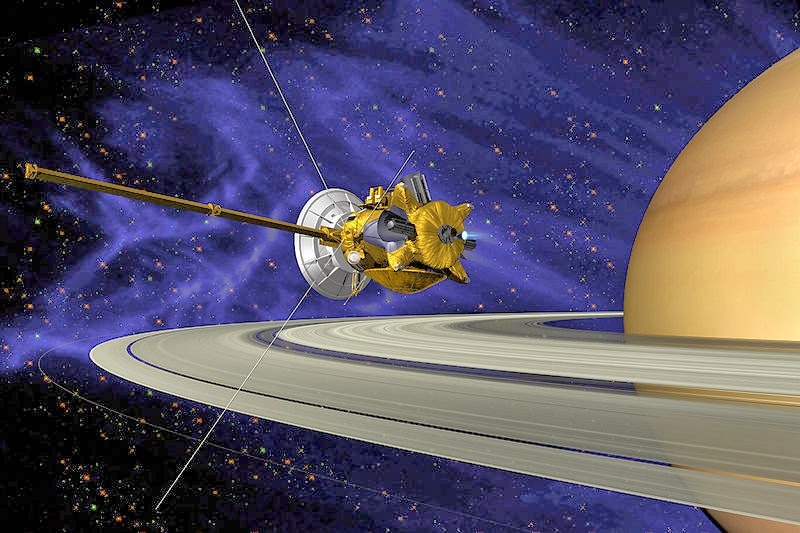## Friday, April 4, 2014

### The Numerology of the PlanetsCassini Satellite around Saturn | Cassini = 3+1+19+19+9+14+9 = 74

Standard Method
• Sun = 19+21+14 = 54, reduces to 9
• Mercury = 13+5+18+3+21+18+25 = 103, reduces to 4
• Venus = 22+5+14+21+19 = 81, reduces to 9
• Earth = 5+1+18+20+8 = 52, reduces to 7
• Mars = 13+1+18+19 = 51, reduces to 6
• Jupiter = 10+21+17+8+20+5+18 = 99, reduces to 18, reduces to 9
• Saturn = 19+1+20+21+18+14 = 93, reduces to 12, reduces to 3
• Uranus = 21+18+1+14+21+19 = 94, reduces to 13, reduces to 4
• Neptune = 14+5+16+20+21+14+5 = 95, reduces to 14, reduces to 5
• Pluto = 16+12+21+20+15 = 84, reduces to 12, reduces to 3
• Discovered 84-years after Neptune
Pythagorean Method
• Sun = 1+3+5 = 9
• Mercury = 4+5+9+3+3+9+7 = 40, reduces to 4
• Venus = 4+5+5+3+1 = 18, reduces to 9
• Earth = 5+1+9+2+8 = 25, reduces to 7
• Mars = 4+1+9+1 = 15, reduces to 6
• Jupiter = 1+3+7+9+2+5+9 = 36, reduces to 9
• Saturn = 1+1+2+3+9+5 = 21, reduces to 3
• Uranus = 3+9+1+5+3+1 = 22, reduces to 4
• Neptune = 5+5+7+2+3+5+5 = 32, reduces to 5
• Pluto = 7+3+3+2+6 = 21, reduces to 3
Most interesting of all, is that Saturn and Pluto have the numerology of the religion of Thelema, established by Aleister Crowley.  You can read more about that here.  Jupiter, the largest of the planets, also has fascinating numerology of 99 which reduces to 9.

By looking at the history of Aleister Crowley's Thelema courtesy of Wikipedia, we can learn a thing or two about coding words into numbers, and more complex system that date back centuries.
"The number 93 is of great significance in Thelema, a religious philosophy founded by English author and occultist Aleister Crowley in 1904 with the writing of The Book of the Law (also known as Liber AL vel Legis).
The central philosophy of Thelema is in two phrases from Liber AL: "Do what thou wilt shall be the whole of the Law" and "Love is the law, love under will." The two primary terms in these statements are "Will" and "Love", respectively. In the Greek language, they are Thelema (Will) and Agape (Love). Using the Greek technique of isopsephy, which applies a numerical value to each letter, the letters of each of these words add to 93:
Thelema"
Θελημα 93 Θ (Theta) 9 ε (Epsilon) 5 λ (Lambda) 30 η (Eta) 8 μ (Mu) 40 α (Alpha) 1
Agapé
Αγαπη 93 Α (Alpha) 1 γ (Gamma) 3 α (Alpha) 1 π (Pi) 80 η (Eta) 8
The Cassini Satellite is currently orbiting Saturn, and as of today, April 4, 2014, has made a potential discovery on one of Saturn's moons, saying that water has possibly been found there.  You can read more about there here.  The numerology of Cassini is also interesting, matching that of Jesus, Cross and Occult.
• Jesus = 10+5+19+21+19 = 74
• Cross = 3+18+15+19+19 = 74
• Occult = 15+3+3+21+12+20 = 74
• Cassini = 3+1+19+19+9+14+9 = 74